emphasis to the concept of inequality. A meaningful comparison between two quantities can be set up if they are related in some way, even though the relationship may not be one of equality. The expression "number sentence" is often used to describe a general relationship which may be either an equality or an inequality."> InequalitiesCustom SearchINEQUALITIES Modern mathematical thought gives considerable emphasis to the concept of inequality. A meaningful comparison between two quantities can be set up if they are related in some way, even though the relationship may not be one of equality. The expression "number sentence" is often used to describe a general relationship which may be either an equality or an inequality. If the number sentence states an equality, it is an EQUATION; if it states an inequality, it is an INEQUATION. ORDER PROPERTIES OF REAL NUMBERS The idea of order, or relative rank according to size, is based upon two intuitive concepts: "greater than" and �less than." Mathematicians use the symbol > to represent "greater than" and the symbol < to represent �less than." For example, the inequation stating that 7 is greater than 5 is written in symbols as follows: 7 > 5 The inequation stating that x is less than 10 is written as follows: x < 10 A "solution" of an inequation involving a variable is any number which may be substi right member. For example, the inequation x < 10 has many solutions. All negative numbers zero, and all positive numbers less than 10, may be substituted for x successfully. These solutions comprise a set of numbers, called the SOLUTION SET. The SENSE of an inequality refers to the direction in which the inequality symbol points. For example, the following two inequalities have opposite sense:         7 > 5         10 < 12 PROPERTIES OF INEQUALITIES Inequations may be manipulated in accordance with specific operational rules, in a manner similar to that used with equations. Addition The rule for addition is as follows: If the same quantity is added to both members of an inequation, the result is an inequation having the same sense as the original inequation. The following examples illustrate this: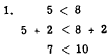The addition of 2 to both members does not change the sense of the inequation.The addition of -3 to both members does not change the sense of the inequation. Addition of the same quantity to both members is a useful method for solving inequations. In the following example, 2 is added to both members in order to isolate the x term on the left:Multiplication The rule for multiplication is as follows: If both members of an inequation are multiplied by the same positive quantity, the sense of the resulting inequation is the same as that of the original inequation. This is illustrated as follows:Multiplication of both members by 2 does not change the sense of the inequation.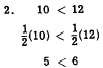Multiplication of both members by 1/2 does not change. the sense of the inequation. Notice that example 2 illustrates division of both members by 2. Since any division can be rewritten as multiplication by a fraction, the multiplication rule is applicable to both multiplication and division. Multiplication is used to simplify the solution of inequations such as the following: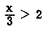Multiply both members by 3: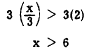Sense Reversal If both sides of an inequation are multiplied or divided by the same negative number, the sense of the resulting inequation is reversed. This is illustrated as follows:Sense reversal is useful in the solution of an inequation in which the variable is preceded by a negative sign, as follows:         2 - x < 4 Add -2 to both members to isolate the x term: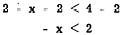Multiply both members by - 1:                 x > -2 Practice problems. Solve each of the following inequations :Integrated Publishing, Inc. - A (SDVOSB) Service Disabled Veteran Owned Small Business